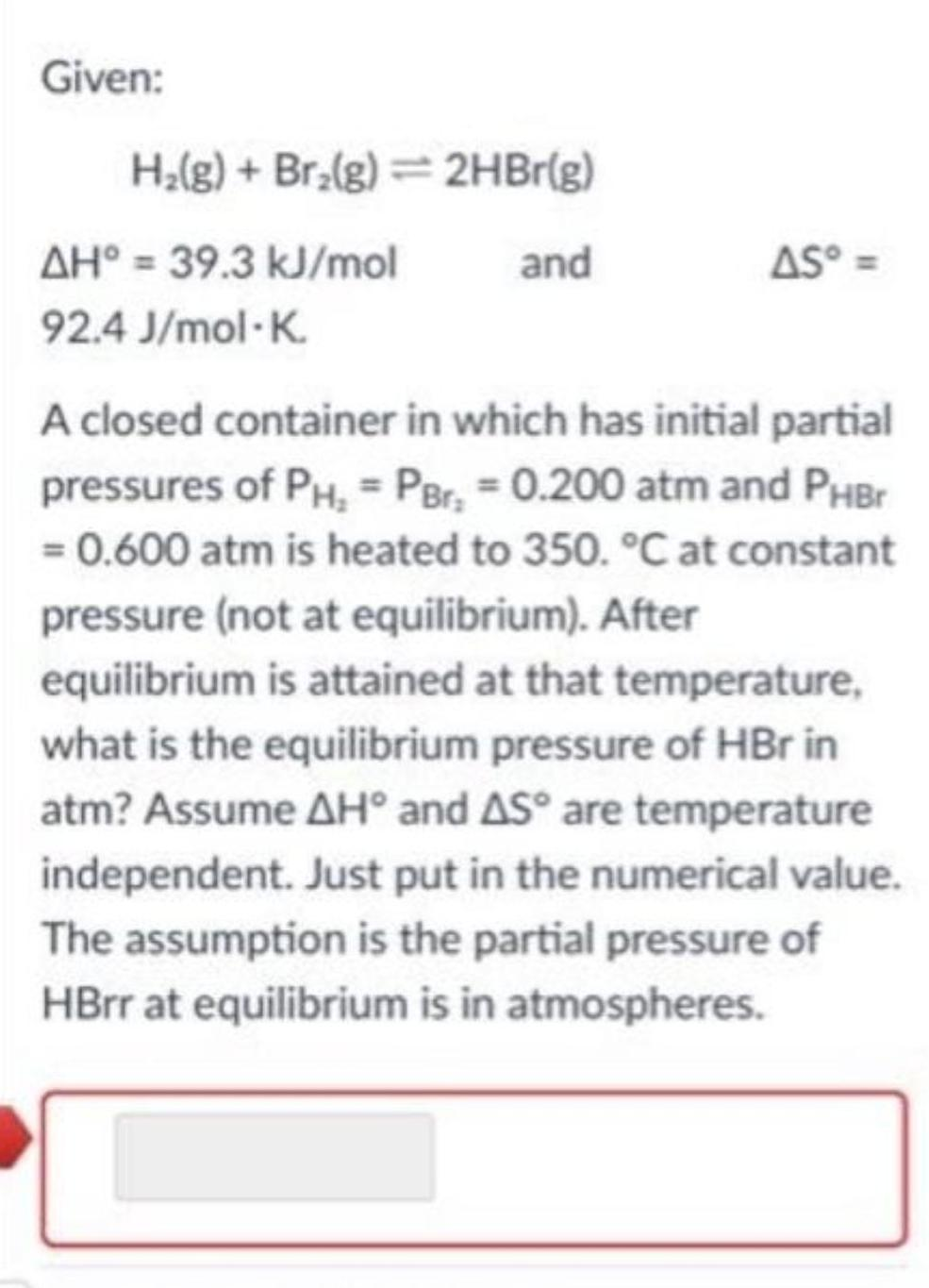Question:

# Given: H₂(g) + Br₂(g) = 2HBr(g) and ΔH° = 39.3 kJ/mol ΔS° =

Last updated: 8/8/2022Given: H₂(g) + Br₂(g) = 2HBr(g) and ΔH° = 39.3 kJ/mol ΔS° = 92.4 J/mol-K. A closed container in which has initial partial pressures of PH₂ = PBr₂ = 0.200 atm and PHBr = 0.600 atm is heated to 350. °C at constant pressure (not at equilibrium). After equilibrium is attained at that temperature, what is the equilibrium pressure of HBr in atm? Assume ΔH and ΔS are temperature independent. Just put in the numerical value. The assumption is the partial pressure of HBrr at equilibrium is in atmospheres.